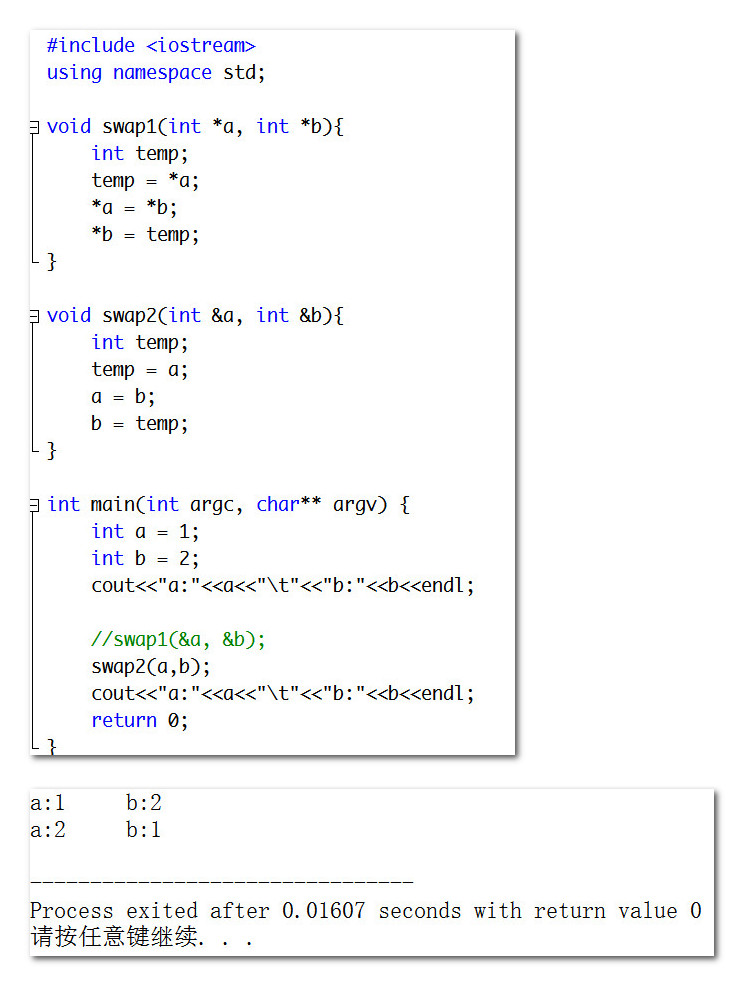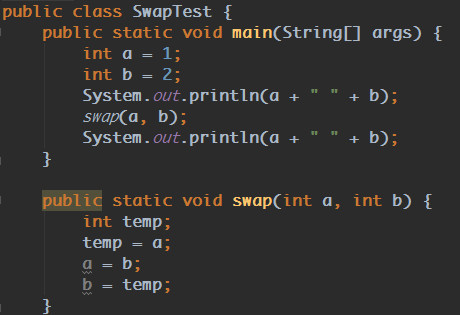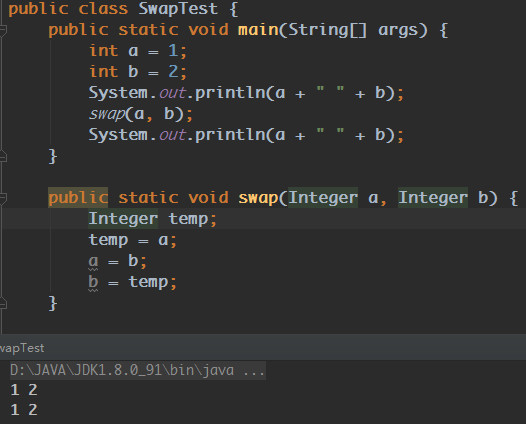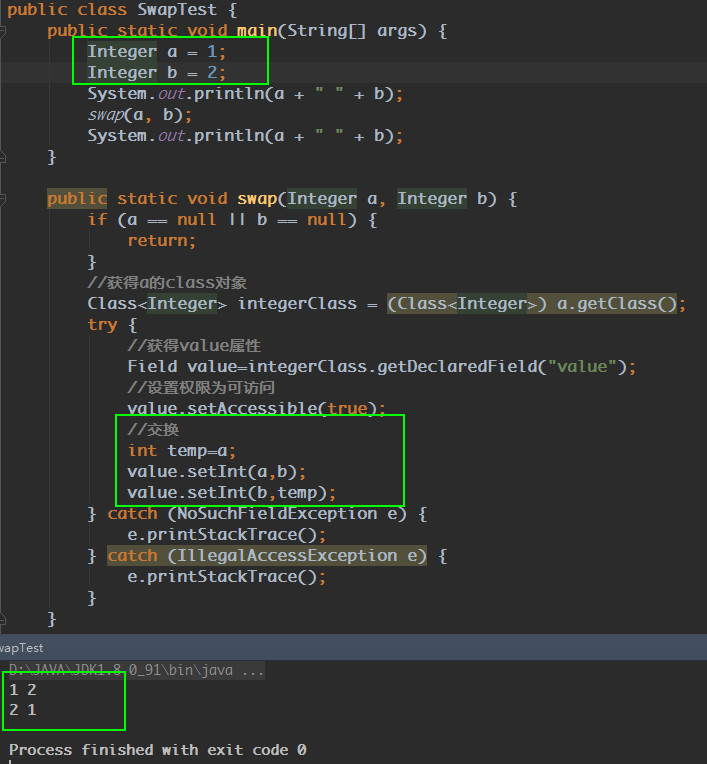# Java中的swap

## C/C++中的swap

void swap(int *a, int *b)
{
int temp;
temp = a;
a = b;
b = temp;
}


C++里面可以使用指针也可以使用引用来实现：

void swap1(int *a, int *b)
int temp;
temp = *a;
*a = *b;
*b = temp;
}

void swap2(int &a, int &b){
int temp;
temp = a;
a = b;
b = temp;
}## Java中的swap

Java中不能直接操作指针变量，int、double、float等基本类型函数传参的时候都是值传递，也就是传入函数的只是原来变量的一个副本，所以在函数中交换是副本，并达不到交换的目的。import java.lang.reflect.Field;

public class SwapTest {
public static void main(String[] args) {
Integer a = 1;
Integer b = 2;
System.out.println(a + " " + b);
swap(a, b);
System.out.println(a + " " + b);
}

public static void swap(Integer a, Integer b) {
if (a == null || b == null) {
return;
}
//获得a的class对象
Class<Integer> integerClass = (Class<Integer>) a.getClass();
try {
//获得value属性
Field value=integerClass.getDeclaredField("value");
//设置权限为可访问
value.setAccessible(true);
//交换
int temp=a;
value.setInt(a,b);
value.setInt(b,temp);
} catch (NoSuchFieldException e) {
e.printStackTrace();
} catch (IllegalAccessException e) {
e.printStackTrace();
}
}

//    public static void swap(Integer a, Integer b) {
//        Integer temp;
//        temp = a;
//        a = b;
//        b = temp;
//    }

//    public static void swap(int a, int b) {
//        int temp;
//        temp = a;
//        a = b;
//        b = temp;
//    }

}



## 总结

C/C++中swap是通过传递变量地址(指针或引用)来交换变量地址中的值。Java对程序员屏蔽了变量地址的概念，为的是减少指针误用。

1. 原始类型(char，int，double等）都是通过直接拷贝变量值传参；
2. 对象类型都是通过引用拷贝(跟C++中引用不同)传参，通过该引用能够更改其指向的对象内部值，但是更改该引用值，仅对函数内部可见，函数外部的实参依然没有改变；

01-02327302-163481
12-101469
11-091万+
09-21779
10-242万+
02-1262
02-1319
02-16216
02-2480
02-12176
01-135460
10-118271
01-1354
02-1276
02-2169
06-102万+
©️2020 CSDN 皮肤主题: 大白 设计师:CSDN官方博客